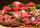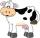Drying herbs

Herbs lose 75% weight By drying. How many fresh herbs do you have to collect if you want to make 1.5 kg of dried herbs?

Result

x =  6 kg

Solution:Leave us a comment of this math problem and its solution (i.e. if it is still somewhat unclear...):Be the first to comment!To solve this verbal math problem are needed these knowledge from mathematics:

Our percentage calculator will help you quickly calculate various typical tasks with percentages. Do you have a linear equation or system of equations and looking for its solution? Or do you have quadratic equation?

Next similar math problems:

1. Meat losesMeat loses 18% of its weight by smoking. How much raw meat butcher used to manufacture 35 kilos of smoked?
2. Sales offThe price has decreased by 20%. How many percents do I have to raise the new price to be the same as before the cut?
3. Frameworks is badCalculate how many percent will increase the length of an HTML document, if any ASCII character unnecessarily encoded as hexadecimal HTML entity composed of six characters (ampersand, grid #, x, two hex digits and the semicolon). Ie. space as: &#x20;
4. PercentsHow many percents is 900 greater than the number 750?
5. Highway repairThe highway repair was planned for 15 days. However, it was reduced by 30%. How many days did the repair of the highway last?
6. SummerjobThe temporary workers planted new trees. Of the total number of 500 seedlings, they managed to plant 426. How many percents did they meet the daily planting limit?
7. Cinema ticketsCinema sold 180 tickets this Thursday, which is 20%. Monday 14%, Tuesday 6%, Wednesday 9%, Friday 24%, Saturday 12%, and Sunday 15%. How many tickets were sold per week?
8. CowsAgricultural cooperative has increased the number of housed cows by 14% to 285 units. By how many cows increased agricultural cooperative the number of cows?
9. CacaoCacao contains 34% filling. How many grams of filling are in 130 g cacao.
10. Jane classWhen asked how many students are in class, Jane said, if we increase the number of students in our class by hundred % and then add half the number of students, we get 100. How many students are in Jane's class?
11. GirlsThe children's competition was attended by 63 girls, which is 30% of all children's participants. How many children attended this competition?
12. Fifth of the numberThe fifth of the number is by 24 less than that number. What is the number?
13. Reducing numberReducing the an unknown number by 28.5% we get number 243.1. Determine unknown number.
14. CompetitorsIn the first round of slalom fell 15% of all competitors and in the second round another 10 racers. Together, 40% of all competitors fell. What was the total number of competitors?
15. Percentages52 is what percent of 93?
16. Finding the baseFind unknown base of percent: 12.5 percent of what = 16 ?
17. Profit gainIf 5% more is gained by selling an article for Rs. 350 than by selling it for Rs. 340, the cost of the article is: